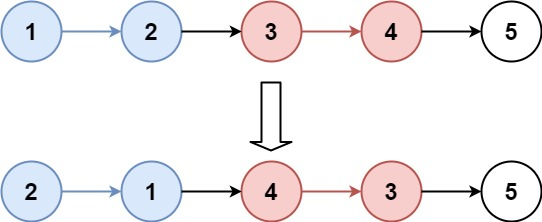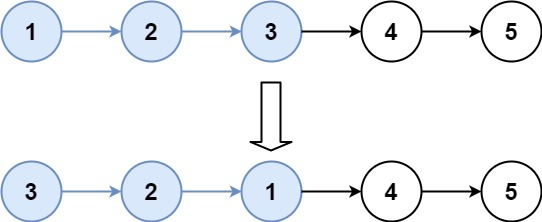### LeetCode• ㊗️
• 大家
• offer
• 多多！

## Problem

Given the head of a linked list, reverse the nodes of the list k at a time, and return the modified list.

k is a positive integer and is less than or equal to the length of the linked list. If the number of nodes is not a multiple of k then left-out nodes, in the end, should remain as it is.

You may not alter the values in the list’s nodes, only nodes themselves may be changed.

Example 1:Input: head = [1,2,3,4,5], k = 2
Output: [2,1,4,3,5]


Example 2:Input: head = [1,2,3,4,5], k = 3
Output: [3,2,1,4,5]


Constraints:

• The number of nodes in the list is n.
• 1 <= k <= n <= 5000
• 0 <= Node.val <= 1000

## Code

/**
* public class ListNode {
*     int val;
*     ListNode next;
*     ListNode(int x) { val = x; }
* }
*/
class Solution {
public ListNode reverseKGroup(ListNode head, int k) {
int count = k;

// 移动到末尾
while(count != 0 && end != null){
end = end.next;
count--;
}

if(count == 0){
end = reverseKGroup(end, k);
for(int i = 0; i < k; i++){